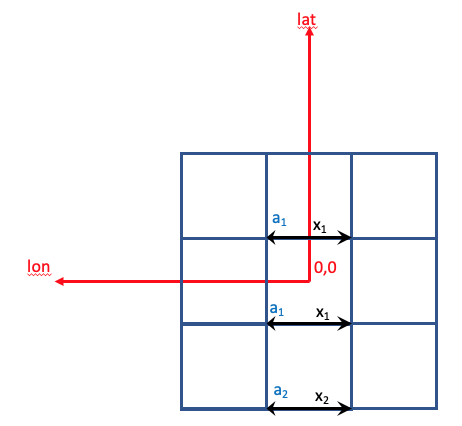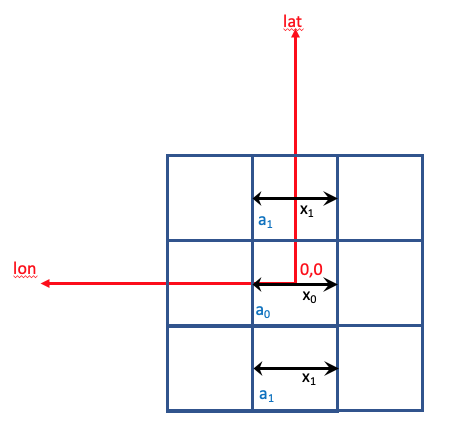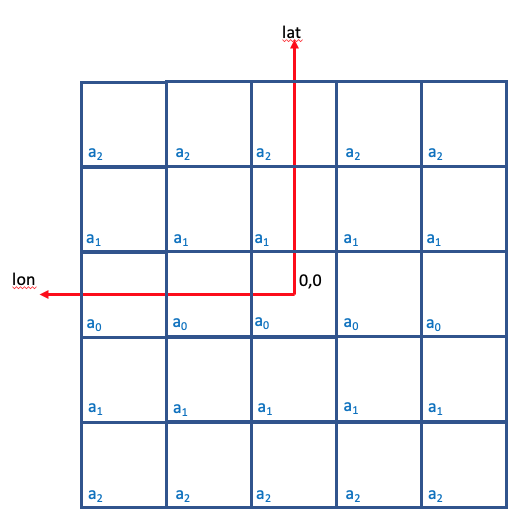{{ message }}

# Fix symmetry issue in solid angle calculation for WcsGeom#2035

Merged
merged 7 commits into from Feb 18, 2019
Merged

# Fix symmetry issue in solid angle calculation for WcsGeom#2035

merged 7 commits into from Feb 18, 2019

## Conversation

###watsonjj commented Feb 15, 2019

 Currently the solid angle calculation for a `WcsGeom` is not symmetric about the origin. This is a result of calculating the separation along the bin edge instead of along the bin centre. The text was updated successfully, but these errors were encountered:
``` Create test that requires symmetric solid angle calculation ```
``` 43b3f86 ```

###watsonjj commented Feb 15, 2019

 I will explain the problem, and what is causing it:In the image above I have created a Wcs Map centred on the origin with three bins in each axis. Currently the dx values for the solid angle calculation are calculated along the bottom of the pixels as shown in the above figure. Due to the great circle separations of the coordinate frame, the separation between the lon coordinates of the pixel edges reduces as you increase/decrease in lat. As the bottom of the pixels is symmetric for the top two pixels, they have the same x separation. They will therefore result in the same solid angle. Whereas the bottom pixel will have a different solid angle value, as x2 is measured at a further distance along lat from the origin. The problem I see with this is the solid angle measured for each pixel is not symmetric about the origin. I would have expected the top and bottom pixel to have the same solid angle value, as they both have the same distance along the lat axis.If one instead measures the separation along the centre of the pixel, as show above, then the calculation is symmetric about the origin, and the solid angles come out as expected.The above image shows the expected area distribution across pixels.

``` Calculate separations through the centre of the pixel ```
``` 14893e0 ```

###registerrier left a comment • edited

Looks good. It is nice to have spotted that.

How significant is the change in the solid angle results for typical test cases we have implemented. I imagine there should be some change in many gammapy.cube tests, no?

 ylo_xlo = lon[..., :-1, :-1], lat[..., :-1, :-1] ylo_xhi = lon[..., :-1, 1:], lat[..., :-1, 1:] yhi_xlo = lon[..., 1:, :-1], lat[..., 1:, :-1] # TODO: Calculate actual solid angle between two great circles?

###registerrier Feb 15, 2019

A priori I think that one can use great circle only for very specific `WCS` projections, e.g. for the Plate-Carrée or `CAR` projection. But for most other projections the axes do not follow great circles e.g. Hammer-Aitoff `AIT` or tangential `TAN`.

So it is possible to use exact formulae for some specific projections, but not in general.

###watsonjj Feb 15, 2019

Okay. Shall I remove this TODO?

``` Correct fixed values in tests (change on the order of 0.01%) ```
``` aad5f23 ```

###watsonjj commented Feb 15, 2019

 How significant is the change in the solid angle results for typical test cases we have implemented. I imagine there should be some change in many gammapy.cube tests, no? I have committed the changes required for other tests that had a small (on the order of 0.01%) change in the fixed values

###registerrier left a comment

Thanks @watsonjj !

``` Add references for solid angle computation improvements ```
``` 06c2003 ```

###adonath left a comment

I added two references for the TODO comment on improving the solid angle computation. Thanks @watsonjj for spotting and fixing this issue!

added 3 commits Feb 18, 2019
``` Merge branch 'master' into symmetric_solid_angle ```
``` 74b9212 ```
``` Merge branch 'master' into symmetric_solid_angle ```
``` 6567659 ```
``` Merge remote-tracking branch 'origin/symmetric_solid_angle' into symm… ```
``` f502df7 ```
```…etric_solid_angle

* origin/symmetric_solid_angle:```

###adonath left a comment

Thanks for fixing the merge conflicts, @watsonjj!